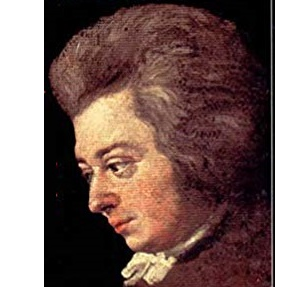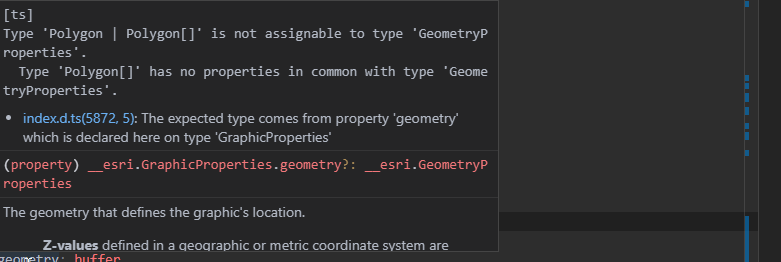# Typescript to Javascript Translation question...

387
3
04-18-2019 01:19 PMRegular Contributor

I'm continuing my quest to pick up some Typescript and have started to 'translate' some JS examples in the ESRI documentation to Typescript. Oftentimes, that's not really much work. But I'm struggling with the following buffer example that came from here:

``````var bufferLayer = new GraphicsLayer();
var pointLayer = new GraphicsLayer();

// [...]

var polySym = {
type: "simple-fill", // autocasts as new SimpleFillSymbol()
color: [140, 140, 222, 0.5],
outline: {
color: [0, 0, 0, 0.5],
width: 2
}
};

// [...]
function createBuffer(event, view) {
// [...]
event.stopPropagation();

// convert screen coordinates to map coordinates
var point = view.toMap({
x: event.x,
y: event.y
});

if (point) {
bufferPoint(point);
}
}

// [...]
function bufferPoint(point) {
// [...]
clearGraphics();

// removes z values from the point when taken from a SceneView.
// GeometryEngine does not support 3D geometries.
point.hasZ = false;
point.z = undefined;

new Graphic({
geometry: point,
symbol: pointSym
})
);

// [...]

var buffer = geometryEngine.geodesicBuffer(point, 560, "kilometers");
new Graphic({
geometry: buffer,
symbol: polySym
})
);
}

// [...]
function clearGraphics() {
pointLayer.removeAll();
bufferLayer.removeAll();
}‍‍‍‍‍‍‍‍‍‍‍‍‍‍‍‍‍‍‍‍‍‍‍‍‍‍‍‍‍‍‍‍‍‍‍‍‍‍‍‍‍‍‍‍‍‍‍‍‍‍‍‍‍‍‍‍‍‍‍‍‍‍‍‍‍‍‍‍‍‍‍‍‍‍‍‍‍‍‍‍‍‍‍‍‍‍‍‍‍‍‍‍‍‍‍‍‍‍‍‍‍‍‍‍‍‍‍‍‍‍‍‍‍‍‍‍‍‍‍‍‍‍‍‍‍‍‍‍‍‍``````

My attempt to convert this to Typescript looks like this:

``````let bufferLayer = new GraphicsLayer();
let pointLayer = new GraphicsLayer();

function createBuffer(event:any, view:MapView) {
event.stopPropagation();

let point = view.toMap({
x: event.x
y: event.y
});

if (point) {
bufferPoint(point);
}
}

function bufferPoint(point:Point) {
clearGraphics();

new Graphic({
geometry: point,
})
);

let buffer = geometryEngine.geodesicBuffer(point, 1, "miles");

let polySym = {
type: "simple-fill", // autocasts as new SimpleFillSymbol()
color: [140, 140, 222, 0.5],
outline: {
color: [0, 0, 0, 0.5],
width: 2
}
};

new Graphic({
geometry: buffer,
symbol: polySym
})
);    ‍‍‍‍‍‍‍‍‍‍‍‍‍‍‍‍‍‍‍‍‍‍‍‍‍‍‍‍‍‍‍‍‍‍‍‍‍‍‍‍‍‍‍‍‍‍‍‍‍‍‍‍‍‍‍‍‍‍‍‍‍‍‍‍‍‍‍‍‍‍‍‍‍‍‍‍‍‍‍‍‍‍‍‍‍‍‍‍‍‍‍‍‍‍‍‍‍‍‍‍‍‍‍‍‍‍‍‍‍‍‍‍‍‍‍‍‍‍‍‍‍‍‍‍‍‍‍‍‍‍‍‍‍‍‍‍‍‍‍‍‍‍‍‍‍‍‍‍‍‍‍‍‍‍‍‍‍‍‍‍‍‍‍‍‍‍‍‍‍‍‍‍‍‍‍‍‍‍‍‍‍‍‍‍‍‍‍‍``````

This works for the point, which is added to the point graphics layer if I comment out the last section ("let bufferLayer,,,,). But it doesn't work for the buffer. When I try to compile, it screams:So somewhere I must be forgetting to correctly declare the right type ? geometry property appears to be type __esri.GraphicProperties, and buffer __esri.Polygon. The ESRI example was for API v11 ... so has something changed? How do I make this jive?

3 RepliesFrequent Contributor II

TypeScript doesn't jive well with autocasting, so if you want to be proper about it, you need to new up all the autocast properties like new SimpleFillSymbol(), new SimpleLineSymbol(), new Color() and so on.

Or you can add an as any to your constructor arguments to force it.byEsri Frequent Contributor

According to the documentation the result of geodesicBuffer can be either a polygon geometry or an array of polygon geometries.

`` let buffer = geometryEngine.geodesicBuffer(point, 1, "miles");``

Because you are passing in one geometry you know the output will be a single polygon. So one thing to try would be to do something like this (note that I haven't tested this code)

const buffer = geometryEngine.geodesicBuffer(point, 1, "miles") as __esri.Poylgon;

You could also try type guarding as suggested in this article where you check for each of the result options:

https://codingblast.com/typescript-union-types-type-guards-type-aliases/Frequent Contributor II

Scratch my post, I misread the question. Kelly knows what she's doing.